# JP2007292856A - Image forming apparatus, method of calculating amount of consumption of developer, and program - Google Patents

Image forming apparatus, method of calculating amount of consumption of developer, and program Download PDF

## Info

Publication number
JP2007292856A
JP2007292856A JP2006117978A JP2006117978A JP2007292856A JP 2007292856 A JP2007292856 A JP 2007292856A JP 2006117978 A JP2006117978 A JP 2006117978A JP 2006117978 A JP2006117978 A JP 2006117978A JP 2007292856 A JP2007292856 A JP 2007292856A
Authority
JP
Japan
Prior art keywords
weighting factor
means
light beam
corresponding
image
Prior art date
Legal status (The legal status is an assumption and is not a legal conclusion. Google has not performed a legal analysis and makes no representation as to the accuracy of the status listed.)
Pending
Application number
JP2006117978A
Other languages
Japanese (ja)
Inventor
Tatsuo Oyama

Original Assignee
Ricoh Co Ltd

Priority date (The priority date is an assumption and is not a legal conclusion. Google has not performed a legal analysis and makes no representation as to the accuracy of the date listed.)
Filing date
Publication date
Application filed by Ricoh Co Ltd, 株式会社リコー filed Critical Ricoh Co Ltd
Priority to JP2006117978A priority Critical patent/JP2007292856A/en
Publication of JP2007292856A publication Critical patent/JP2007292856A/en
Pending legal-status Critical Current

• 238000004364 calculation methods Methods 0.000 claims description 164
• 230000000875 corresponding Effects 0.000 claims description 113
• 238000000034 methods Methods 0.000 claims description 66
• 230000000737 periodic Effects 0.000 claims description 23
• 230000001276 controlling effects Effects 0.000 claims description 3
• 239000000203 mixtures Substances 0.000 description 3
• 239000003795 chemical substance by application Substances 0.000 description 2

## Images

••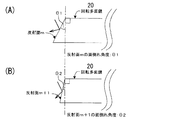•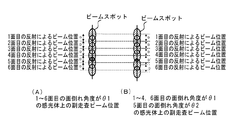•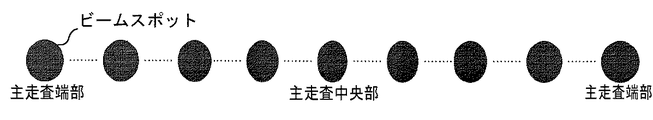•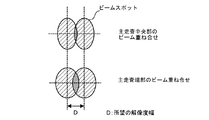•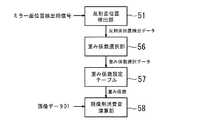•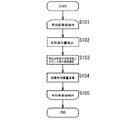•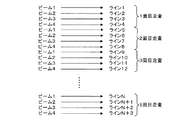•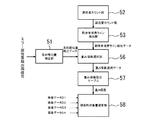•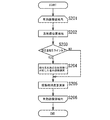•••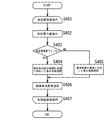•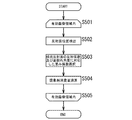•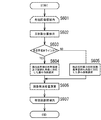•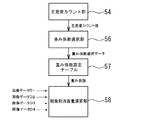•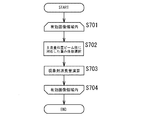•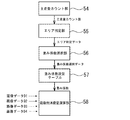•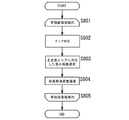•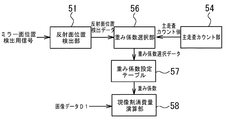•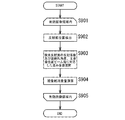•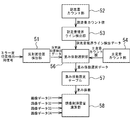•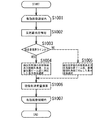•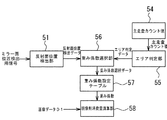•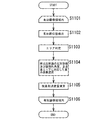•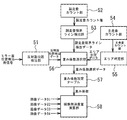•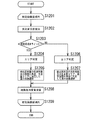## Abstract

<P>PROBLEM TO BE SOLVED: To calculate the consumption amount of a developer with high accuracy, while considering change in exposure state, depending on the characteristics of a main scanning means using a deflector comprising a rotating polygon mirror. <P>SOLUTION: A weight coefficient selector 56 previously acquires a weight coefficient for giving an optimum value which has previously been stored in a weight coefficient setting table, while being related to characteristics, such as coefficient of reflection, surface tilt angle, etc., of a reflective surface, and sets it as a weighting coefficient that a developer consumption arithmetic unit 58 uses for operation. The consumption of the developer is computed, based on the image data used for scan exposure of a photoreceptor and a weighting coefficient varied according to the exposure state, thereby calculating the amount of consumption of the developer with high accuracy. <P>COPYRIGHT: (C)2008,JPO&INPIT

## Description

The present invention relates to an electrophotographic image forming apparatus that scans and exposes a photosensitive member with a light beam emitted from an LD light source that is controlled to be turned on by image data, and develops a generated latent image with toner. The present invention relates to an image forming apparatus provided with means for accurately calculating a toner consumption amount in the image forming process and a toner consumption amount calculating method.

In image forming apparatuses such as printers and copiers that perform image formation by electrophotography, the parts around the developing unit including toner used in the image forming process can be replaced by the user as a replacement part (process cartridge). The method is adopted.
In the process cartridge replacement method, it is necessary to make it possible to replace the cartridge in use at an appropriate time before the use limit approaches and the image quality deteriorates, so the toner consumption is managed.
The toner consumption amount is also managed in order to perform control for stabilizing the image quality such as the image density to be formed.
As described above, the following Patent Documents 1 and 2 can be cited as examples of conventional techniques for managing and controlling the amount corresponding to the toner consumption amount.
In Patent Document 1 (Japanese Patent Laid-Open No. 2005-3904), the number of pixels corresponding to the amount of toner used is integrated from image data of a document, and on the other hand, a patch image developed on a photoconductor is read with an optical device prepared separately. An image forming apparatus including a density control unit that determines a control target value from the obtained read value and the calculated number of pixels is described.
Further, in Patent Document 2 (Japanese Patent Laid-Open No. 2005-4049), in a process cartridge having a reusable process part, print amount information corresponding to the amount printed by an image signal is managed as a history, and the process part An image forming apparatus is described in which the control value of the part is set based on the print amount information at the time of reuse. Here, as the print amount information, count information obtained by counting either the time during which the photosensitive member is exposed or the time during which the photoconductor is not exposed is counted, or the count is obtained by counting the number of dots printed by the image signal. It is described as value information.
JP 2005-3904 A JP 2005-4049 A

However, since the conventional techniques exemplified in Patent Documents 1 and 2 only measure the number of pixels and the exposure time, the exposure beam spreads on the photoconductor and is generated on the photoconductor by the exposure beam. Measurements that take into account variation factors depending on image formation contents such as overlapping of dots are not made, and the consumption of developer cannot be accurately detected and determined.
Therefore, the applicant of the present application previously proposed Japanese Patent Application No. 2005-326650 (hereinafter referred to as “prior art”).
In this prior art, there is a one-to-one correspondence between scan line information of a light emission control signal represented by an array of print dots having a value corresponding to an input image, and one or more dots close to the target dot. Based on the weighting factor corresponding to, it is possible to calculate the amount of developer consumption with high accuracy by performing calculations according to the contents of the image formation, taking into account the overlap between the main scanning and the adjacent dots in the sub-scanning direction. Is described.

By the way, in the image forming method in which the LD is controlled by a light emission control signal represented by an array of dots having a value corresponding to image data, and the emitted light beam is subjected to main / sub two-dimensional scanning and exposure, the main scanning of the light beam is performed. It is common to use main scanning means using a deflector composed of a rotating polygon mirror (polygon mirror) (see FIG. 1).
Since the rotary polygon mirror used here uses each reflection surface for exposure scanning, uniform characteristics are required for each surface, but in practice, variations in the reflection coefficient of each surface are unavoidable. Even if the same amount of beam is emitted from the light source, it is deflected by the reflecting surface having different reflection coefficients, so that the exposure amount due to the beam spot on the photoreceptor varies, and the amount of developer consumed varies depending on the reflecting surface. End up.
Further, the angle of each reflecting surface of the rotary polygon mirror 20 also has a slight error, and as shown in FIG. 2, the angle of inclination of the reflecting surface m and the reflecting surface m + 1 forms different angles θ1 and θ2 for each surface. .
FIG. 3 is a diagram for explaining the influence on the scanning light beam due to the difference in the surface tilt angle. The beam spot row on the (A) side in FIG. 3 shows the positions of the spots projected by the reflecting surfaces when the surface tilt angles θ of the reflecting surfaces are all in the same ideal state. The beam pitch interval in all the spot rows in the sub-scanning direction is D, and no error occurs.
On the other hand, the beam spot row on the (B) side of FIG. 3 shows the position of each spot projected by each reflecting surface when the surface tilt angle θ of the reflecting surface is partially different. Since the beam pitch interval of the spot row in the sub-scanning direction is different only in the plane tilt angle of the fifth surface, the spot projected by the fifth surface is shifted from the position where the spot should be, and this shift causes the sub-scan of this portion. The beam pitch interval becomes D ′, which is different from the interval D of other spots. Due to the positional deviation of the spot, the beam overlapping portion shown by the hatched portion in FIG. 3 (B) is different from the case of FIG. 3 (A), and the consumption amount of the developer is different.

Further, since the scanning optical system constituting the main scanning means linearly scans the light beam projected on the mirror surface by the rotation of the polygon mirror, the beam spot diameter scanned on the exposure surface depends on the position in the main scanning direction. Has varying characteristics.
That is, as shown in FIG. 4, the main scanning width of the beam spot at the end position tends to be larger than the main scanning width of the beam spot at the center position on the exposure surface, and the beam spot diameter varies across the exposure surface. A constant exposure state cannot be obtained.
As shown in FIG. 5, the variation of the beam spot diameter causes the area of the overlapping range of the beams in the main scanning direction (hatched portion in FIG. 5) to vary, and the developer consumption depends on the position in the main scanning direction. The amount will be different.
In addition, even if the fluctuation factors described above are suppressed and fluctuations in the beam spot diameter are eliminated, when the resolution is increased by narrowing the beam pitch interval, the area where the beams overlap increases, and the developer development amount tends to differ. Appears more prominently.
As described above, the exposure state varies depending on the characteristics of the main scanning means using the deflector composed of a rotating polygon mirror. However, the above-described prior art and prior art methods consider the variation of the exposure state. Therefore, it is impossible to calculate the developer consumption with high accuracy.
The present invention has been made in view of the above problems in the prior art and the prior art developer consumption calculation methods, and the problem to be solved is that of the main scanning means using a deflector comprising a rotating polygon mirror. In consideration of fluctuations in the exposure state depending on the characteristics, it is to realize more accurate calculation of developer consumption in the image forming method.

In the invention of claim 1, lighting can be controlled, a light source that outputs a light beam at the time of lighting, and a light beam output from the light source is deflected in a main scanning direction by a deflector including a rotating polygon mirror, A main scanning means for periodically scanning; a photoconductor exposed by a light beam periodically scanned by the main scanning means; a sub-scanning means for displacing the photoconductor in a direction crossing the main scanning direction of the light beam; Image exposure means for controlling the lighting of the light emission source with image data, exposing the photosensitive member with an output light beam, and forming a latent image on the photosensitive member, taking the operation and timing of the main scanning means and the sub-scanning means; In the image forming apparatus having a developing means for generating a visible image by attaching a developer to the latent image formed on the photoconductor by the image exposure means, the image forming apparatus has a value corresponding to image data used for lighting control. Based on scanning line data for lighting control represented by an array of dots, and a weighting factor for calculating developer consumption set corresponding to the difference in the positional relationship between the target dot and adjacent dots in the scanning line data Obtained by the consumption amount calculating means for calculating the consumption amount of the developer, the characteristic information acquiring means for acquiring the information indicating the characteristics of the main scanning means used for the scanning exposure of the photoconductor, and the characteristic information acquiring means. According to the present invention, there is provided a weighting factor setting unit for setting a weighting factor used for the consumption amount calculating unit according to the characteristics of the main scanning unit. By doing so, the above-described problems are solved.

According to a second aspect of the present invention, in the image forming apparatus according to the first aspect, when the characteristic information acquisition unit performs periodic scanning of the light beam, the reflecting surface of the rotary polygon mirror used for each periodic scanning is identified and specified. The weight coefficient setting means sets a weight coefficient corresponding to the corresponding reflection coefficient from the weight coefficients prepared in advance corresponding to the reflection coefficient. In this way, the above-mentioned problems are solved.
According to a third aspect of the present invention, in the image forming apparatus according to the first aspect, when the characteristic information acquisition unit performs periodic scanning of the light beam, the reflecting surface of the rotary polygon mirror used for each periodic scanning is specified and specified. Means for obtaining a surface tilt angle corresponding to each of the reflected surfaces, wherein the weighting factor setting means is a weighting factor corresponding to the corresponding surface tilting angle from a weighting factor prepared in advance corresponding to the surface tilting angle. It is a means for setting the above, and by doing so, the above-mentioned problems are solved.
According to a fourth aspect of the present invention, in the image forming apparatus according to the first aspect, when the characteristic information acquisition unit performs periodic scanning of the light beam, the reflecting surface of the rotary polygon mirror used for each periodic scanning is identified and specified. Means for obtaining a reflection coefficient and a surface tilt angle corresponding to each of the reflected surfaces, and the weighting factor setting means is configured to obtain a corresponding reflection coefficient from a weight coefficient prepared in advance corresponding to the reflection coefficient and the surface tilt angle. And a means for setting a weighting coefficient according to the angle of inclination, and the above-described problem is solved by doing so.

According to a fifth aspect of the present invention, in the image forming apparatus according to the first aspect, the characteristic information acquisition means is generated in the main scanning direction of the spot diameter of the light beam for exposing the photosensitive member in each periodic scanning of the light beam. The weighting factor setting unit sets a weighting factor corresponding to the corresponding variation from a weighting factor prepared in advance corresponding to the variation occurring in the main scanning direction of the spot diameter of the light beam. In this way, the above-mentioned problems are solved.
According to a sixth aspect of the present invention, in the image forming apparatus according to the first aspect, when the characteristic information acquisition unit performs periodic scanning of the light beam, the reflection surface of the rotary polygon mirror used for each periodic scanning is identified and specified. A means for acquiring a variation that occurs in the main scanning direction of the spot diameter of the light beam that exposes the photosensitive member in each periodic scanning of the light beam at the same time as acquiring the reflection coefficient and the surface tilt angle corresponding to each of the reflected surfaces. The weighting coefficient setting means includes a reflection coefficient, a surface inclination angle, and a weighting coefficient prepared in advance corresponding to a reflection coefficient, a surface inclination angle, and a variation that occurs in the main scanning direction of the spot diameter of the light beam. It is a means for setting a weighting factor in accordance with the fluctuation that occurs in the main scanning direction of the spot diameter of the light beam. By doing so, the above-mentioned problems are solved.
According to a seventh aspect of the present invention, in the image forming apparatus according to the fifth or sixth aspect, the fluctuation that occurs in the main scanning direction of the spot diameter acquired by the characteristic information acquisition unit divides the main scanning direction into areas and divides the area. It is characterized in that it is given by a method in which one value is associated with each area, and the above-described problem is solved by doing so.

According to an eighth aspect of the present invention, there is provided a program for causing a program to function as the consumption calculation unit, the characteristic information acquisition unit, and the weighting factor setting unit in the image forming apparatus according to any one of the first to seventh aspects. The above-mentioned problems are solved by being driven by a computer mounted on the computer.

The invention according to claim 9 is a main scanning step in which the light beam output from the light source is deflected in the main scanning direction by the main scanning means using a deflector composed of a rotating polygon mirror, and periodically scanned. Taking the timing of sub-scanning process, main scanning and sub-scanning, which are displaced in the direction crossing the main scanning direction, the lighting source is controlled to be turned on by image data, the photosensitive member is exposed with the output light beam, and the latent image is formed on the photosensitive member. Development for calculating the consumed developer by the image forming process comprising the image exposure process for forming the image and the development process for attaching the developer to the latent image formed on the photoconductor to generate a visible image. In the method for calculating the amount of consumption of the agent, the scanning line data for lighting control represented by an array of dots having values corresponding to the image data used for lighting control, and the positions of the target dot and the neighboring dots in the scanning line data A consumption amount calculating step for calculating a consumption amount of the developer based on a weight coefficient for calculating the developer consumption amount set corresponding to the difference in the relationship, and the deflection means used for scanning exposure of the photosensitive member. Performing a characteristic information acquisition step of acquiring information representing the characteristic, and a weighting factor setting step of setting a weighting factor used in the consumption calculation step according to the characteristic of the deflecting means acquired by the characteristic information acquisition step. The above-described problems are solved by doing so.

The invention of claim 10 is the consumption calculation method according to claim 9, wherein the characteristic information acquisition step specifies the reflecting surface of the rotary polygon mirror used for each periodic scan when the optical beam is periodically scanned. A step of obtaining a reflection coefficient corresponding to each of the identified reflection surfaces, wherein the weight coefficient setting step means calculates a weight coefficient corresponding to the corresponding reflection coefficient from a weight coefficient prepared in advance corresponding to the reflection coefficient. This is a setting step, and the above-described problem is solved by doing so.
The invention of claim 11 is the consumption calculation method according to claim 9, wherein when the characteristic information acquisition step causes the light beam to periodically scan, the reflecting surface of the rotary polygon mirror used for each periodic scan is specified, It is a step of acquiring a surface tilt angle corresponding to each of the specified reflecting surfaces, the weight coefficient setting step means according to the corresponding surface tilt angle from a weight coefficient prepared in advance corresponding to the surface tilt angle This is a step of setting a weighting coefficient, and the above-described problem is solved by doing so.
According to a twelfth aspect of the present invention, in the consumption calculation method according to the ninth aspect, the characteristic information acquisition step is performed in the main scanning direction of the spot diameter of the light beam that exposes the photosensitive member in each periodic scanning of the light beam. The weighting factor setting step means obtains a weighting factor corresponding to the corresponding variation from a weighting factor prepared in advance corresponding to the variation occurring in the main scanning direction of the spot diameter of the light beam. This is a setting step, and the above-described problem is solved by doing so.

According to the present invention, the reflection coefficient, the surface tilt angle, and the light of the reflecting surface in the light beam deflecting means using the rotating polygon mirror, which are not considered in the developer consumption calculation methods proposed in the prior art and the prior art. By taking account of fluctuations in the spot diameter of the beam, the consumption can be calculated with higher accuracy. As a result, because of the necessity for accuracy, the conventionally used sensor for detecting the remaining amount of developer is not required, and the running time can be extended by supplying a more optimal amount of developer. In addition, it provides more accurate information on developer usage status and developer replenishment and replacement timing, and can supply the optimum amount of developer without supplying too much or too little developer. As a result, the quality of the image to be formed is improved, and the detection accuracy of the amount of waste toner is improved.
In addition, since the main scanning direction is divided into areas and the representative values are made to correspond to the divided areas, it is possible to reduce the scale of the means for storing and managing the fluctuation value generated in the main scanning direction of the spot diameter. (Claim 7)
In addition, since a function for calculating consumption, obtaining characteristic information of the main scanning means, and setting a weighting coefficient is provided by the program, the function realizing means can be easily configured.

Embodiments according to the present invention will be described below.
FIG. 1 shows a schematic configuration of an image forming apparatus of the present invention.
The image forming apparatus shown in FIG. 1 is an apparatus for forming an image by a so-called electrophotographic method in which a photosensitive member is exposed with a periodically scanned light beam and a formed latent image is developed with a developer (toner). The principal part relevant to invention is shown.
The light beam output system in the apparatus shown in FIG. 1 includes a write controller 50, a laser driver 15, and an LD 10.
The write control unit 50 outputs control data for turning on the LD 10 as a light emitting source at a predetermined timing based on image data received from the preceding image processing unit (not shown) when a print output is requested. Input to the drive unit 15. The lighting control data output from the writing control unit 50 is data represented by an array of dots having values corresponding to the image data. The LD driver 19 receives the lighting control data and sends a drive signal to the LD 10. Then, the LD 10 is turned on. The light emitted from the LD 10 is output as a light beam. The LD 10 uses an LD array having a plurality of light sources when simultaneous writing with a plurality of beams is possible.
The light beam scanning system has, as a deflector, a rotating polygon mirror (polygon mirror) 20 that is rotated by a drive unit 22 having a polygon motor as a drive source. The rotary polygon mirror 20 is periodically rotated by the driving unit (polygon motor) 22 and periodically scans the output light beam from the LD 10 projected onto the mirror surface that is sequentially switched by the rotation. The light beam periodically scanned by the light beam scanning system scans the photosensitive surface of the photosensitive drum 30 at a constant speed in the main scanning direction, and generates a line image as a latent image.
In addition, it is necessary to acquire data for specifying the reflecting surface of the rotary polygon mirror used for scanning the line image of each cycle as data necessary for calculating the developer consumption amount described later, and drive the signal for this purpose. The data is output from the unit 22 to the write control unit 50. The polygon motor of the drive unit 22 can be controlled, and the rotation speed of the polygon motor is changed when changing the resolution.
The synchronization detection sensor 40 detects a light beam in the main scanning optical path and generates a synchronization signal. The writing control unit 50 aligns the image position in the main scanning direction on the photosensitive drum 30 by writing each line image at a fixed timing with reference to the synchronization signal from the synchronization detection sensor 40. The scanning position of the light beam is detected by the count value of the main scanning count unit, and is used for the above-described writing timing control and calculation of the developer consumption amount described later.

The photosensitive drum 30 is rotationally driven by a motor, and the rotation position of the light beam on the photosensitive drum 30 is changed by this rotation. That is, the light beam is sub-scanned, and the position of the main scanning line moves in the sub-scanning direction. Since the main scanning line interval changes when the rotational speed of the photoconductive drum 30 varies, a constant rotational speed is required during image writing. Note that the resolution can be changed by changing the rotational speed of the photosensitive drum 30.
The writing control unit 50 generates a sub-scanning gate signal for determining an image position in the sub-scanning direction with reference to the generated writing start signal in synchronization with the transfer paper conveyance, and generates a line image in the gate period. Write. The line position is detected by the count value of the sub-scanning counting unit, and is used for writing timing control and calculation of developer consumption amount to be described later.
The write control unit 50 includes a CPU for executing write control and processing related to writing, and a storage unit for storing various programs necessary for processing of control data and the like, and the CPU drives the program. Realize control functions. The program used here includes a program for calculating and managing a developer consumption amount to be described later, in addition to the writing control.
Further, a predetermined region of the photosensitive drum 30 is exposed by main / sub scanning of the light beam modulated by the image data, and the formed latent image is developed by a developer (toner). That is, a developer (toner) is attached to the latent image through a developing device (not shown) that acts on the exposure surface of the photosensitive drum 30 to generate a visible image. Note that the electrophotographic image forming process itself such as formation of a latent image by exposure of the photosensitive drum 30, development with toner, and transfer of a toner image on the photosensitive member to a recording medium is an existing technology. Here, the description is omitted.

The present invention proposes a technique for determining the amount of developer (toner) consumed when image formation is performed by an electrophotographic method as in the apparatus illustrated in FIG.
Here, the method employed for determining the consumption amount of the developer is basically determined based on image data used for scanning exposure of the photosensitive drum 30. That is, since a line image is formed by using data represented by an array of dots having a value corresponding to the image data as a writing signal, development is performed with a certain functional relationship with the exposure energy given by the written dots. Assuming that the agent is consumed.
It is known that this functional relationship between the developer consumption amount and the exposure energy differs depending on whether or not the exposure areas of one scan line image and another scan line image overlap each other in one scan line image.
Therefore, in order to calculate the total consumption of the image for which print output is requested, the degree of overlap of the exposure regions is detected from the image data of the target image, and the total amount of energy in the regions that do not overlap with the total amount of energy in the overlapping regions is calculated. First, a calculation operation is required in which each is multiplied by a different weighting factor and then added, and the calculated sum is obtained as a correct consumption amount of the target image. Here, in order to estimate the correct consumption, the weighting coefficient must be experimentally obtained in advance.
Note that the method of calculating the developer consumption amount by the above operation can be performed based on the method in the prior art (Japanese Patent Application No. 2005-326650) described above. Here, the description is omitted.

The feature of the method of the present invention depends on the characteristics of the main scanning means using a deflector composed of a rotating polygonal mirror, as described with reference to FIGS. This makes it possible to obtain a more accurate amount of developer consumption in consideration of fluctuations in the exposure state.
In the following, an embodiment relating to the calculation of the consumption amount of the developer having this characteristic will be shown.
Here, as a variation factor depending on the characteristics of the main scanning unit using a deflector composed of a rotating polygon mirror, which affects the calculation result of the consumption amount of the developer,
(1) Reflection coefficient of rotating polygon mirror
(2) Angle of tilt of reflecting surface of rotating polygon mirror
(3) Consider factors for fluctuations in the main scanning direction of the spot diameter of the light beam on the exposure surface.
The above factors (1) to (3) may occur variably due to these factors alone or in combination.
Further, as will be described in detail later, when an LD array having a plurality of light sources is used as a light source capable of simultaneously writing with a plurality of beams, between adjacent lines having the same period and adjacent lines having different scanning periods. The influence on the developer consumption due to different exposure conditions is taken into consideration as a variable factor.
The main variations of the developer consumption calculation method that can be applied in response to the case where these variable factors are involved in different forms are shown in “Embodiment 1” to “Embodiment 12” below.

“Embodiment 1”
This embodiment shows a means for calculating the correct consumption amount corresponding to the fact that the reflection coefficient of the rotary polygon mirror 20 is different for each reflection surface and becomes a factor that causes the calculated developer consumption amount to be incorrect. Is.
Here, when calculating the developer consumption amount, the reflecting surface of the rotary polygon mirror 20 used for writing the image is specified, and the setting of the weighting coefficient used for the calculation for calculating the developer consumption amount of the image is performed. By changing according to the reflection coefficient of the specified reflection surface, the difference in the reflection coefficient does not become an error.
FIG. 6 shows the configuration of the developer consumption calculation means of this embodiment as a functional block.
The developer consumption calculation means shown in FIG. 6 includes a developer consumption calculation unit 58, a reflection surface position detection unit 51, a weighting coefficient selection unit, and a means for setting a weighting factor in the developer consumption calculation unit 58. 56 and a weight coefficient setting table 57.
The weighting factor setting table 57 obtains the relationship between the reflection coefficient of the rotary polygon mirror 20 and the weighting factor in advance by experiment, and stores and manages the obtained data as a table in a storage medium such as NV (nonvolatile) RAM. The data stored as a table may correspond to a method in which a weighting factor is directly referred to from a reflecting surface position that specifies which reflecting surface of the rotary polygon mirror 20, or the weighting factor is determined via the reflecting factor. You may make it respond | correspond to the system to refer.
The reflection surface position detection unit 51 is used for image writing based on a position detection signal indicating the rotation position of the mirror surface sent from the polygon motor drive unit 22 that rotates the rotary polygon mirror 20. It is specified which surface the reflecting surface of the rotary polygon mirror 20 is.
The weighting factor selection unit 56 performs an operation of selecting a weighting factor with reference to the weighting factor setting table 57 based on the reflecting surface position data detected by the reflecting surface position detection unit 51. The weighting coefficient obtained by referring to the weighting coefficient setting table 57 by the weighting coefficient selection data is set in the developer consumption amount calculation unit 58.
Since this embodiment uses an LD that emits one beam as the light source, as shown in FIG. 6, the rotating polygon mirror is scanned for each scan line with respect to the image data D1 input to the consumption calculation unit 58. Since the 20 reflecting surfaces are changed, the weighting coefficient is also reset for each line.

With reference to FIG. 7, the flow of the developer consumption calculation processing performed by the developer consumption calculation means (FIG. 6) will be described.
When a print output is requested, the writing control unit 50 starts image writing control in accordance with an image forming execution instruction, and scans and exposes the photosensitive drum 30 with a light beam. As part of this writing control, the flow of the developer consumption calculation process shown in FIG. 7 is executed.
According to the flow shown in FIG. 7, first, it is confirmed that the writing is in the effective image area (step S101), and the calculation process of the developer consumption amount for the first line image is started.
First, the reflecting surface is detected as to which reflecting surface of the rotary polygon mirror 20 used for writing the image of the first line (step S102). Here, from the position detection signal indicating the rotation position of the mirror surface sent from the polygon motor drive unit 22 by the reflection surface position detection unit 51, for example, if there are 6 surfaces, which surface is 1 to 6. judge.
Next, a weighting factor corresponding to the reflection coefficient of the specified reflecting surface is selected (step S103). The weighting factor selection unit 56 refers to the weighting factor setting table 57 in which the reflecting surface and the weighting factor are associated with each other based on the data specifying the reflecting surface detected by the reflecting surface position detecting unit 51, A weighting coefficient corresponding to the reflection coefficient is selected, and the obtained weighting coefficient is set in the developer consumption amount calculation unit 58.
Next, the developer consumption amount calculation unit 58 calculates the developer consumption amount for the image data D1 of the first line by calculation using the set weight coefficient (step S104).
This calculation of the developer consumption amount is repeated for each line up to the image of the last line in the effective image area for the data used for writing the line image. Accordingly, the processing flow is confirmed to be within the effective area (step S105), and if this confirmation can be made, the process returns to step S102 and the calculation of the developer consumption is repeated.
If it is confirmed in step S105 that the effective area has been exceeded, this processing flow ends.
In the present embodiment, as described above, even when the reflection coefficient of the rotary polygon mirror 20 is different for each reflection surface, it becomes possible to adjust the developer consumption amount to a correct value by changing the weighting coefficient, The intended purpose can be achieved.

“Embodiment 2”
This embodiment has the same purpose as the first embodiment in that the correct consumption is calculated when the reflection coefficient of the rotating polygon mirror 20 is different for each reflection surface, but an LD array is used as the light source. This corresponds to the case of scanning with a plurality of beams.
When scanning with a plurality of beams, lines 1 to 4 are simultaneously written in one scanning of the photosensitive surface by one reflecting surface of the rotary polygon mirror 20, as shown in the example of four beams in FIG. The lines 5 to 8 are simultaneously written by the four-beam scanning by the next reflecting surface. In this scanning method, the exposure condition differs on the reflecting surface, and this difference becomes an element that causes the developer consumption amount to be calculated to be erroneous. Therefore, means for calculating the correct consumption amount corresponding to this variable element is shown. Is.
Here, when calculating the developer consumption amount, the reflecting surface of the rotary polygon mirror 20 used for image writing is specified, and boundary lines in a plurality of line images simultaneously written on one reflecting surface are detected. By changing the setting of the weighting coefficient used in the calculation for calculating the developer consumption of the image to each reflecting surface and changing the coefficient according to each surface, the difference between the variable elements does not become an error. To do.
FIG. 9 shows the configuration of the developer consumption calculating means of this embodiment as a functional block.
The developer consumption calculating means shown in FIG. 9 is based on the configuration shown in the first embodiment (FIG. 6) as an additional configuration for dealing with switching of the reflecting surface of the rotary polygon mirror 20 as a sub-scanning counting unit. 52 and a sub-scanning boundary line detection unit 53.
The sub-scan count unit 52 outputs a sub-scan count value that determines the writing position of the line image in the sub-scan direction.
The sub-scanning boundary line detection unit 53 performs sub-scanning based on the sub-scanning count value passed from the sub-scanning counting unit 52 and the number of lines (4 lines in the illustrated example) simultaneously written by scanning with one reflecting surface. Detect boundary lines.
Note that the weighting factor selection unit 56 and the weighting factor setting table 57 in the developer consumption calculating means shown in FIG. 9 can be basically configured in the same manner as in FIG. 6, but whether or not they are sub-scanning boundary lines. Since the selection operation of the weighting coefficient is performed according to the sub-scanning boundary line detection data indicating such, naturally, the corresponding configuration needs to be changed.
In addition, the developer consumption calculation unit 58 also writes a plurality of lines simultaneously on one reflecting surface of the rotary polygon mirror 20, switches to the next reflecting surface, and simultaneously writes a plurality of lines. It is assumed that the arithmetic processing is performed in units of the number of lines (in this example, units of four image data D1 to D4).

With reference to FIG. 10, the flow of the developer consumption calculation process performed by the developer consumption calculation means (FIG. 9) will be described.
When a print output is requested, the writing control unit 50 starts image writing control in accordance with an image forming execution instruction, and scans and exposes the photosensitive drum 30 with a light beam. As part of this writing control, the flow of processing for calculating the developer consumption shown in FIG. 10 is executed.
According to the flow shown in FIG. 10, first, it is confirmed that the writing is in the effective image area (step S201), and the calculation process of the developer consumption amount for the first line image to be written is started.
A weighting coefficient selection operation is performed in the main scanning direction (step S204). That is, the weighting factor selection unit 56 does not refer to the weighting factor setting table 57 while the sub-scanning boundary line detection data is not output. Accordingly, when the sub-scanning boundary line is detected, one weighting factor obtained from the weighting factor setting table 57 is not changed as a weighting factor used for each image of four lines to be simultaneously written on one reflecting surface, and the developer consumption calculating unit 58.
Next, the developer consumption amount calculation unit 58 calculates the consumption amount of the developer for each of the four image data D1 to D4 on the first surface by calculation using a set weight coefficient (step S205).
This calculation of the developer consumption is repeated for every four lines that are simultaneously written on one reflecting surface up to the image of the last line in the effective image area for the data used for writing the line image. Accordingly, the processing flow is confirmed to be within the effective area (step S206), and if this confirmation can be made, the process returns to step S202 again to repeat the calculation of the developer consumption.
If it is confirmed in step S206 that the effective area has been exceeded, this processing flow ends.
In the present embodiment, as described above, even when the reflection coefficient of the rotary polygon mirror 20 is different for each reflection surface and writing of a plurality of beams is performed simultaneously on one reflection surface, the developer consumption is achieved by changing the weight coefficient. The amount can be adjusted to the correct value and the intended purpose can be achieved.

“Embodiment 3”
This embodiment corresponds to the fact that the angle of inclination (see FIGS. 2 and 3) of the rotary polygon mirror 20 is different for each reflecting surface, and if it is constant, the calculated consumption of the developer becomes an element that is erroneous. It shows the means for calculating the quantity.
Here, when calculating the developer consumption amount, the reflecting surface of the rotary polygon mirror 20 used for writing the image is specified, and the setting of the weighting coefficient used for the calculation for calculating the developer consumption amount of the image is performed. By changing according to the surface tilt angle of the specified reflecting surface, the difference in the surface tilt angle is prevented from becoming an error.
The developer consumption calculating means of the present embodiment can be configured in the same manner as FIG. 6 shown as means when the reflection coefficients of the reflecting surfaces of the rotary polygon mirror 20 are different in the first embodiment.
However, in the first embodiment, the weighting factor setting table 57 in FIG. 6 has a relationship between the reflection coefficient and the weighting factor, but in this embodiment, it has a relationship between the surface tilt angle and the weighting factor. That is, in the weighting factor setting table 57, the relationship between the surface tilt angle of the rotary polygon mirror 20 and the weighting factor is obtained in advance by experiments, and the obtained data is stored as a table in a storage medium such as NVRAM and managed.

FIG. 11 shows a flow of processing for calculating the developer consumption performed by the developer consumption calculating means of the present embodiment.
When a print output is requested, the writing control unit 50 starts image writing control in accordance with an image forming execution instruction, and scans and exposes the photosensitive drum 30 with a light beam. As part of this writing control, the flow of the developer consumption calculation process shown in FIG. 11 is executed.
According to the flow shown in FIG. 11, first, it is confirmed that the writing is in the effective image area (step S301), and the calculation process of the developer consumption amount for the first line image is started.
First, the reflecting surface is detected as to which reflecting surface of the rotary polygon mirror 20 used for writing the image of the first line (step S302). Here, from the position detection signal indicating the rotation position of the mirror surface sent from the polygon motor drive unit 22 by the reflection surface position detection unit 51, for example, if there are 6 surfaces, which surface is 1 to 6. judge.
Next, a weighting coefficient corresponding to the specified tilt angle of the reflecting surface is selected (step S303). The weighting factor selection unit 56 refers to the weighting factor setting table 57 in which the reflecting surface and the weighting factor are associated with each other based on the data specifying the reflecting surface detected by the reflecting surface position detecting unit 51, A weighting factor corresponding to the surface tilt angle is selected, and the obtained weighting factor is set in the developer consumption amount calculation unit 58.
Next, the developer consumption amount calculation unit 58 calculates the developer consumption amount for the image data D1 of the first line by calculation using the set weight coefficient (step S304).
The calculation of the developer consumption is repeated for each line until the final line image in the effective image area is sequentially applied to the data used for writing the line image. Accordingly, the processing flow is confirmed to be within the effective area (step S305), and if this confirmation can be made, the process returns to step S302 and the calculation of the developer consumption is repeated.
If it is confirmed in step S305 that the effective area has been exceeded, the process flow ends.
In the present embodiment, as described above, even when the surface tilt angle of the rotary polygon mirror 20 is different for each reflecting surface, it is possible to adjust the developer consumption amount to a correct value by changing the weighting factor. , Can achieve the intended purpose.

“Embodiment 4”
This embodiment has the same purpose as that of the third embodiment in that the correct consumption is calculated when the angle of inclination of the rotary polygon mirror 20 is different for each reflecting surface, but an LD array is used as the light source. This corresponds to the case of scanning with a plurality of beams.
When scanning with a plurality of beams, lines 1 to 4 are simultaneously written in one scanning of the photosensitive surface by one reflecting surface of the rotary polygon mirror 20, as shown in the example of four beams in FIG. The lines 5 to 8 are simultaneously written by the four-beam scanning by the next reflecting surface. In this scanning method, the exposure condition becomes non-uniform depending on the angle of inclination of the reflecting surface, and this causes an error in the calculated developer consumption. Means for calculating are shown.
Here, when calculating the developer consumption amount, the reflecting surface of the rotary polygon mirror 20 used for image writing is specified, and boundary lines in a plurality of line images simultaneously written on one reflecting surface are detected.
By changing the setting of the weighting coefficient used in the calculation for calculating the consumption amount of the developer according to the tilt angle of each surface, the difference between the fluctuation elements does not become an error for the detected boundary line image. Like that.
Note that a value corresponding to the reference plane tilt angle is set as the weighting coefficient applied to images other than the boundary line.

The developer consumption calculating means of the present embodiment can be configured in the same manner as in FIG. 9 shown as means when the surface tilt angles of the reflecting surfaces of the rotary polygon mirror 20 are different in the second embodiment.
However, in the second embodiment, the weighting factor setting table 57 in FIG. 9 has a relationship between the reflection coefficient and the weighting factor, but in this embodiment, it has a relationship between the surface tilt angle and the weighting factor. That is, in the weighting factor setting table 57, the relationship between the surface tilt angle of the rotary polygon mirror 20 and the weighting factor is obtained in advance by experiments, and the obtained data is stored as a table in a storage medium such as NVRAM and managed.
Here, when an emission source having a constant distance between LD light sources is used, such as an LD array that outputs a plurality of beams, in an example in which four beams are written in one scan, as shown in FIG. The beam pitch intervals in the beam spot row on the (A) side where the plane tilt angles θ 1 are all in the same ideal state are all D, and no error will occur if a weighting factor corresponding to this plane tilt angle is applied. . If the surface tilt angle θ 1 that is the beam pitch interval D is a reference, a weighting coefficient for the surface tilt angle is stored in the weighting coefficient setting table 57 as a reference value.
On the other hand, the beam spot column shown in FIG. 12B when the surface tilt angle θ of the reflecting surface is different between the reflecting surface m (θ 1 ) and the reflecting surface m + 1 (θ 2 ) is the sub-scanning boundary line. The beam pitch interval between the spots is D ′, which is different from the spot interval D other than the boundary line. Due to the positional deviation of the spot, the overlapped portion of the beam at the sub-scanning boundary has a different result from the case of FIG. 12A. If the same weighting coefficient as in the beam pitch interval D is used here, the developer consumption An error will occur in the quantity.
Therefore, the weighting factor for guiding the correct consumption relative tilt angle theta 1 and theta 2 when the beam pitch becomes D'is stored in the weight coefficient setting table 57.

With reference to FIG. 13, the flow of the developer consumption calculation process performed by the developer consumption calculation means (FIG. 9) will be described.
When a print output is requested, the writing control unit 50 starts image writing control in accordance with an image forming execution instruction, and scans and exposes the photosensitive drum 30 with a light beam. As part of this writing control, the flow of the developer consumption calculation process shown in FIG. 13 is executed.
According to the flow shown in FIG. 13, first, it is confirmed that the writing is in the effective image area (step S401), and the calculation process of the developer consumption amount for the first line image to be written is started. In the following description, the case where the number of lines simultaneously written on one reflecting surface is four will be described as an example. First, which reflecting surface is the reflecting surface of the rotary polygon mirror 20 used for writing four lines on the first surface. Whether there is a reflection surface is detected (step S402). Here, from the position detection signal indicating the rotation position of the mirror surface sent from the polygon motor drive unit 22 by the reflection surface position detection unit 51, for example, if there are 6 surfaces, which surface is 1 to 6. judge.
Next, a weighting coefficient corresponding to the specified tilt angle of the reflecting surface is selected. However, here, since four lines of images are written on the same reflecting surface, the sub-scanning boundary line is detected, and different weighting factors are selected for the sub-scanning boundary line and the other lines.
For this reason, it is checked whether or not it is a sub-scanning boundary line (step S403). A selection operation is performed (step S404). That is, the weighting factor selection unit 56 uses the weight used for the image according to the difference in the plane tilt angle between the reflecting surfaces (the plane tilt angles θ 1 and θ 2 in FIG. 12B) with respect to the sub-scanning boundary line. The coefficients are extracted from the weight coefficient setting table 57 and set in the developer consumption amount calculation unit 58.
On the other hand, when it is not the sub-scanning boundary line (step S403-NO), the selection operation of the weight coefficient corresponding to the reference plane tilt angle is performed (step S405). That is, the weighting factor selection unit 56 sets a reference weighting factor value in the developer consumption amount calculation unit 58 for lines other than the sub-scanning boundary line.
Next, the developer consumption amount calculation unit 58 calculates the consumption amount of the developer for each of the four image data D1 to D4 on the first surface by calculation using the set weight coefficient (step S406).
This calculation of the developer consumption amount is repeated for every four lines that are simultaneously written on one reflecting surface up to the image of the last line in the effective image area for the data used for writing the line image. Accordingly, the processing flow is confirmed to be within the effective area (step S407), and if this confirmation can be made, the process returns to step S402 again to repeat the calculation of the developer consumption.
If it is confirmed in step S407 that the effective area has been exceeded, the process flow ends.
In the present embodiment, as described above, even when the surface tilt angle of the rotary polygon mirror 20 is different on each reflecting surface and a plurality of beams are simultaneously written on one reflecting surface, the developer is changed by changing the weighting coefficient. The consumption amount can be adjusted to a correct value, and the intended purpose can be achieved.

“Embodiment 5”
In the first embodiment and the second embodiment, the means corresponding to the case where the difference in the reflection coefficient and the surface tilt angle on each reflecting surface of the rotary polygon mirror 20 that causes an error in calculating the developer consumption amount occurs independently. However, in this embodiment, a means for calculating a correct consumption amount corresponding to a case where these error factors occur in combination is shown.
The developer consumption calculating means of the present embodiment can be configured in the same manner as FIG. 6 shown as means when the reflection coefficients of the reflecting surfaces of the rotary polygon mirror 20 are different in the first embodiment.
However, in the first embodiment, the weighting coefficient setting table 57 in FIG. 6 has a relationship between the reflection coefficient and the weighting coefficient. However, in this embodiment, the weighting coefficient data and the data obtained by combining the reflection coefficient and the surface tilt angle are combined. It is related. That is, in the weighting coefficient setting table 57, the relationship between the weighting coefficient and the composite data of the reflection coefficient and the surface tilt angle of the rotary polygon mirror 20 is obtained in advance by experiments, and the obtained data is stored in a storage medium such as NVRAM as a table. ,to manage.

FIG. 14 shows a flow of processing for calculating the developer consumption performed by the developer consumption calculating means of this embodiment.
When a print output is requested, the writing control unit 50 starts image writing control in accordance with an image forming execution instruction, and scans and exposes the photosensitive drum 30 with a light beam. As part of this writing control, the flow of processing for calculating the developer consumption shown in FIG. 14 is executed.
According to the flow shown in FIG. 14, first, it is confirmed that the writing is in the effective image area (step S501), and the calculation process of the developer consumption amount for the first line image is started.
First, the reflecting surface is detected as to which reflecting surface of the rotary polygon mirror 20 used for writing the image of the first line (step S502). Here, from the position detection signal indicating the rotation position of the mirror surface sent from the polygon motor drive unit 22 by the reflection surface position detection unit 51, for example, if there are 6 surfaces, which surface is 1 to 6. judge.
Next, a weighting factor corresponding to the combined data of the reflection coefficient and the surface tilt angle of the specified reflecting surface is selected (step S503). The weighting factor selection unit 56 sets the weighting factor by associating the weighting factor with the composite data of the reflection factor and the surface tilt angle of the reflecting surface based on the data specifying the reflecting surface detected by the reflecting surface position detecting unit 51. With reference to the table 57, a weighting coefficient corresponding to the reflection coefficient and surface tilt angle of the reflecting surface is selected, and the obtained weighting coefficient is set in the developer consumption amount calculation unit 58.
Next, the developer consumption amount calculation unit 58 calculates the developer consumption amount for the image data D1 of the first line by calculation using the set weight coefficient (step S504).
This calculation of the developer consumption amount is repeated for each line up to the image of the last line in the effective image area for the data used for writing the line image. Accordingly, the processing flow is confirmed to be within the effective area (step S505), and if this confirmation can be made, the process returns to step S502 and the calculation of the developer consumption is repeated.
If it is confirmed in step S505 that the effective area has been exceeded, this processing flow ends.
In the present embodiment, as described above, even when the reflection coefficient and the surface tilt angle of the rotary polygon mirror 20 are different on each reflection surface, the developer consumption amount can be adjusted to a correct value by changing the weight coefficient. It is possible to achieve the intended purpose.

“Embodiment 6”
This embodiment has the same purpose as that of the fifth embodiment in that the correct consumption is calculated when the reflection coefficient and the surface tilt angle of the rotary polygon mirror 20 are different for each reflection surface. This corresponds to the case where scanning is performed with a plurality of beams using an array.
When scanning with a plurality of beams, lines 1 to 4 are simultaneously written in one scanning of the photosensitive surface by one reflecting surface of the rotary polygon mirror 20, as shown in the example of four beams in FIG. The lines 5 to 8 are simultaneously written by the four-beam scanning by the next reflecting surface. In this scanning method, the exposure condition becomes non-uniform depending on the reflection coefficient of the reflecting surface and the surface tilt angle, and becomes an element that misleads the developer consumption to be calculated. It shows the means for calculating the correct consumption.
Here, when calculating the developer consumption amount, the reflecting surface of the rotary polygon mirror 20 used for image writing is specified, and boundary lines in a plurality of line images simultaneously written on one reflecting surface are detected.
For the detected image of the boundary line, the setting of the weighting coefficient used in the calculation for calculating the developer consumption amount is changed according to the reflection coefficient and the surface tilt angle of each surface, so that the difference between the variable elements is Avoid errors.
It should be noted that values corresponding to the reflection coefficient of each surface and the reference surface tilt angle are set as the weighting factors applied to images other than the boundary lines.

The developer consumption calculating means of the present embodiment can be configured in the same manner as in FIG. 9 shown as means when the surface tilt angles of the reflecting surfaces of the rotary polygon mirror 20 are different in the second embodiment.
However, in the second embodiment, the weighting factor setting table 57 in FIG. 9 has a relationship between the reflection coefficient and the weighting factor, but in the present embodiment, it has a relationship between the reflection coefficient and the surface tilt angle and the weighting factor. That is, in the weighting coefficient setting table 57, a relationship between data obtained by combining the reflection coefficient of the rotary polygon mirror 20 and the surface tilt angle and the weighting coefficient is obtained in advance by experiments, and the obtained data is used as a table to store a storage medium such as NVRAM. Store and manage.
Here, when an emission source having a constant distance between the LD light sources is used, such as an LD array that outputs a plurality of beams, in an example in which writing of four beams is performed in one scan, as shown in FIG. The beam pitch intervals in the beam spot row on the (A) side where the plane tilt angles θ 1 are all in the same ideal state are all D, and at this plane tilt angle, the reflection coefficient of the combined reflecting surface changes. If the corresponding weighting factor is applied, no error occurs. If the surface tilt angle θ 1 that is the beam pitch interval D is a reference, a weighting factor corresponding to a change in reflection coefficient combined with the reference surface tilt angle is stored in the setting table 57 as a reference coefficient value. The
On the other hand, the beam spot column shown in FIG. 12B when the surface tilt angle θ of the reflecting surface is different between the reflecting surface m (θ 1 ) and the reflecting surface m + 1 (θ 2 ) is the sub-scanning boundary line. The beam pitch interval between the spots is D ′, which is different from the spot interval D other than the boundary line. Due to the positional deviation of the spot, the overlapped portion of the beam at the sub-scanning boundary has a different result from the case of FIG. 12A. If the same weighting coefficient as in the beam pitch interval D is used here, the developer consumption An error will occur in the quantity.
Therefore, the weighting factor for guiding the correct consumption relative tilt angle theta 1 and theta 2 and variations of the combination of the reflection coefficient is complexed thereto when the beam pitch becomes D'is, the weight coefficient setting table 57 Stored.

With reference to FIG. 15, the flow of the developer consumption calculation process performed by the developer consumption calculation means (FIG. 9) will be described.
When a print output is requested, the writing control unit 50 starts image writing control in accordance with an image forming execution instruction, and scans and exposes the photosensitive drum 30 with a light beam. As part of this writing control, the flow of the developer consumption calculation process shown in FIG. 15 is executed.
According to the flow shown in FIG. 15, first, it is confirmed that the writing is in the effective image area (step S601), and the calculation process of the developer consumption amount for the first line image to be written is started. In the following description, the case where the number of lines simultaneously written on one reflecting surface is four will be described as an example. First, which reflecting surface is the reflecting surface of the rotary polygon mirror 20 used for writing four lines on the first surface. Whether there is a reflection surface is detected (step S602). Here, from the position detection signal indicating the rotation position of the mirror surface sent from the polygon motor drive unit 22 by the reflection surface position detection unit 51, for example, if there are 6 surfaces, which surface is 1 to 6. judge.
Next, a weighting coefficient corresponding to data obtained by combining the reflection coefficient of the identified reflecting surface and the surface tilt angle is selected. However, here, since four lines of images are written on the same reflecting surface, the sub-scanning boundary line is detected, and different weighting factors are selected for the sub-scanning boundary line and the other lines.
Therefore, it is checked whether or not it is a sub-scanning boundary line (step S603). If it is a sub-scanning boundary line (step S603-YES), the reflection coefficient of the detection reflecting surface and the surface tilt angle are combined. The selection operation of the weighting coefficient corresponding to the set data is performed (step S604). That is, the weighting factor selection unit 56 is a composite data of the difference between the reflection coefficient of the detection surface and the surface tilt angle between the reflective surfaces (the surface tilt angles θ 1 and θ 2 in FIG. 12B) with respect to the sub-scanning boundary line. Accordingly, the weighting factor used for the image is extracted from the weighting factor setting table 57 and set in the developer consumption amount calculation unit 58.
On the other hand, if it is not the sub-scanning boundary line (step S603-NO), a weighting coefficient selection operation corresponding to the reflection coefficient combined with the reference plane tilt angle is performed (step S605). That is, the weighting factor selection unit 56 sets the weighting factor value obtained using the combined surface tilt angle as a reference value for the lines other than the sub-scanning boundary line in the developer consumption amount calculation unit 58.
Next, the developer consumption amount calculation unit 58 calculates the consumption amount of the developer for each of the four image data D1 to D4 on the first surface by calculation using a set weighting coefficient (step S606).
This calculation of the developer consumption amount is repeated for every four lines that are simultaneously written on one reflecting surface up to the image of the last line in the effective image area for the data used for writing the line image. Accordingly, the processing flow is confirmed to be within the effective area (step S607), and if this confirmation can be made, the process returns to step S602 again to repeat the calculation of the developer consumption.
If it can be confirmed in step S607 that the effective area has been exceeded, the process flow ends.
In the present embodiment, as described above, the weighting coefficient is changed even when the reflection coefficient and the surface tilt angle of the rotary polygon mirror 20 are different for each reflection surface and a plurality of beams are simultaneously written on one reflection surface. This makes it possible to adjust the developer consumption amount to a correct value, thereby achieving the intended purpose.

“Embodiment 7”
This embodiment shows a means for calculating the correct consumption amount corresponding to the fluctuation of the spot diameter of the light beam generated on the exposure surface, which is an element that causes the calculated developer consumption amount to be erroneous.
The light beam that is deflected by the reflecting surface of the rotary polygon mirror 20 and scans the exposure surface of the photosensitive drum can be read by using the same dot image data as described with reference to FIGS. According to the calculation method based on the assumption that the beam diameter is constant at the center and the end in the scanning direction because the beam diameter fluctuates, the developer consumption amount is erroneous.
Accordingly, when calculating the developer consumption amount, the setting of the weighting coefficient used for the calculation for calculating the developer consumption amount of the target image is changed according to the image writing position in the main scanning direction, thereby the above-described spot diameter. It is intended to prevent the fluctuation of the error from becoming an error.
FIG. 16 shows the configuration of the developer consumption calculating means of this embodiment as a functional block.
The developer consumption calculation means shown in FIG. 16 includes a developer consumption calculation unit 58 and a main scanning count unit 54 and a weighting factor selection unit 56 as means for setting a weighting factor in the developer consumption calculation unit 58. And a weight coefficient setting table 57.
The weighting factor setting table 57 obtains the relationship between the variation of the spot diameter of the light beam generated in the main scanning direction and the weighting factor, which is a characteristic of the main scanning unit using the rotary polygon mirror 20 by experiment in advance, and is obtained data Are stored in a storage medium such as NV (nonvolatile) RAM as a table and managed. The data stored as a table may correspond to a method of referring to the weighting coefficient from the exposure position.
The main scanning count unit 54 outputs a main scanning count value that determines the writing position of the line image in the main scanning direction.
The weighting factor selection unit 56 performs an operation of selecting a weighting factor with reference to the weighting factor setting table 57 based on the main scanning count value output from the main scanning counting unit 54. The weighting coefficient obtained by referring to the weighting coefficient setting table 57 by the weighting coefficient selection data is set in the developer consumption amount calculation unit 58.
In the example shown in FIG. 16, the developer consumption amount calculation unit 58 performs calculation processing in units of four image data D1 to D4. This is because an LD array that emits a plurality of beams (in this example, four beams) is used as the light source, so that arithmetic processing is performed in units of the number of lines simultaneously written by one reflecting surface of the rotary polygon mirror 20. And Therefore, when an LD that emits one beam is used as the light source, the image data input to the consumption calculation unit 58 is one line, and the reflection surface of the rotary polygon mirror 20 changes for each scanning line. The coefficient is set again for each line.

Referring to FIG. 17, the flow of the developer consumption calculation process performed by the developer consumption calculation means (FIG. 16) will be described.
When a print output is requested, the writing control unit 50 starts image writing control in accordance with an image forming execution instruction, and scans and exposes the photosensitive drum 30 with a light beam. As part of this writing control, the flow of processing for calculating the developer consumption shown in FIG. 17 is executed.
According to the flow shown in FIG. 17, first, it is confirmed that the writing is in the effective image area (step S701), and the calculation of the developer consumption amount for the first line image is started and the calculation of the developer consumption amount is started. A necessary weight coefficient is selected (step S702).
Here, as a weighting coefficient to be applied to scanning line data represented by an array of dots having a value corresponding to image data, a weighting coefficient corresponding to a writing position in the main scanning direction is selected. The weighting factor selection unit 56 associates the writing (exposure) position in the main scanning direction with the weighting factor as the writing position of each dot of the scanning line data based on the main scanning count value obtained by the main scanning counting unit 54. Referring to the weighting factor setting table 57, the weighting factor corresponding to the dot writing position is selected, and the obtained weighting factor is set in the developer consumption calculating unit 58.
Here, since the weighting factor set from the weighting factor setting table 57 is not switched for each reflection surface of the rotary polygon mirror 20, the weighting factor applied to the line image does not change depending on whether the scanning beam is single or plural. Coefficients can be used in common.
Next, the developer consumption calculation unit 58 sets the developer consumption for each line image in units of the number of lines to be written simultaneously (image data D1 for a single beam, D1 to D4 for 4 beams). It is calculated by calculation using the weighting factor (step S703).
This calculation of the developer consumption is sequentially repeated for the data used for writing the line image up to the image of the last line in the effective image area. Accordingly, the processing flow is confirmed to be within the effective area (step S704), and if this confirmation can be made, the process returns to step S702 and the calculation of the developer consumption is repeated.
If it is confirmed in step S704 that the effective area has been exceeded, this processing flow ends.
In the present embodiment, as described above, even when the spot diameter of the light beam generated on the exposure surface fluctuates, it is possible to adjust the developer consumption amount to a correct value by changing the weighting factor. The purpose of the period can be achieved.

“Eighth embodiment”
In this embodiment, as in the seventh embodiment, in the developer consumption calculation means having the purpose of calculating the correct consumption even when the spot diameter of the light beam generated on the exposure surface fluctuates, the weight coefficient setting is performed. The scale of the table 57 is intended to be reduced.
In Embodiment 7, the developer consumption amount calculation weight coefficient per main scanning period is the maximum number of main scanning dots (pixels), and the scale of the weighting coefficient setting table 57 becomes large. By dividing the scanning period into a plurality of areas and sharing the weighting coefficients in the same area, the scale of the weighting coefficient setting table can be reduced.
FIG. 18 shows the configuration of the developer consumption calculating means of this embodiment as a functional block.
The developer consumption calculating means shown in FIG. 18 has an area determination unit 55 as an additional configuration for dealing with area division based on the configuration shown in the seventh embodiment (FIG. 16).
The area determination unit 55 determines the area to which the writing position of the line image in the main scanning direction belongs, based on the main scanning count value from the main scanning count unit 54, and outputs area determination data.
The weighting factor setting table 57 obtains the relationship between the variation of the spot diameter of the light beam generated in the main scanning direction and the weighting factor in advance by the main scanning means using the rotary polygon mirror 20, and based on the obtained data. Although managed by a table, here, the weighting factor is divided within a range of spot diameters that do not hinder the sharing, and the weighting factor corresponding to the determined main scanning area data is stored in the NVRAM as a table.
Note that the weighting factor selection unit 56 in the developer consumption calculating means shown in FIG. 18 can be basically configured in the same manner as in FIG. 16, but performs a weighting factor selection operation according to the area determination data. Of course, corresponding configuration changes are required.

Referring to FIG. 19, the flow of the developer consumption calculation process performed by the developer consumption calculation means (FIG. 18) will be described.
When a print output is requested, the writing control unit 50 starts image writing control in accordance with an image forming execution instruction, and scans and exposes the photosensitive drum 30 with a light beam. As part of this writing control, the flow of the developer consumption calculation process shown in FIG. 19 is executed.
According to the flow shown in FIG. 19, first, it is confirmed that the writing is in the effective image area (step S801), and the processing for calculating the developer consumption for the first line image is started.
First, the area of the line image to be written is determined (step S802). Here, the area determination unit 55 determines the area to which the writing position of the writing dot in the main scanning direction belongs based on the main scanning count value from the main scanning counting unit 54 to obtain area determination data.
Next, on the basis of the area determination data obtained in the previous stage, the weight coefficient corresponding to the dot writing position is selected by referring to the weight coefficient setting table 57 that associates the area data with the weight coefficient, and the obtained weight The coefficient is set in the developer consumption calculating unit 58 (step S803).
Here, since the weighting factor set from the weighting factor setting table 57 is not switched for each reflection surface of the rotary polygon mirror 20, the weighting factor applied to the line image does not change depending on whether the scanning beam is single or plural. Coefficients can be used in common.
Next, the developer consumption calculation unit 58 sets the developer consumption for each line image in units of the number of lines to be written simultaneously (image data D1 for a single beam, D1 to D4 for 4 beams). It is calculated by calculation using the weighting factor (step S804).
This calculation of the developer consumption is sequentially repeated for the data used for writing the line image up to the image of the last line in the effective image area. Accordingly, the processing flow is confirmed to be within the effective area (step S805), and if this confirmation can be made, the process returns to step S802 again to repeat the calculation of the developer consumption.
If it can be confirmed in step S805 that the effective area has been exceeded, the process flow ends.
In the present embodiment, as described above, the intended purpose of reducing the scale of the weighting coefficient setting table can be achieved by introducing the area division method to the line image to be processed.

“Embodiment 9”
In the seventh embodiment, in the calculation of the developer consumption amount, the variation that occurs in the main scanning direction of the spot diameter of the light beam that exposes the photosensitive member is considered as an error factor. In the fifth embodiment, the rotation factor is the error factor. In consideration of the difference in reflection coefficient and surface tilt angle at each reflecting surface of the polygon mirror 20, means corresponding to the case where each occurs separately is shown, but in this embodiment, these error factors are combined and generated. Corresponding to the above, means for calculating the correct consumption is shown.
FIG. 20 shows the configuration of the developer consumption calculating means of this embodiment. As shown in the configuration of FIG. 20, the reflection coefficient on each reflecting surface of the rotary polygon mirror 20 is the same as the configuration of FIG. 16 (Embodiment 7) shown as a means for dealing with fluctuations in the spot diameter of the light beam in the main scanning direction. As a means for coping with the surface tilt angle, a reflecting surface position detector 51 is added.
In addition, the weighting factor selection unit 56 extracts the weighting factor used for the image from the weighting factor setting table 57 and sets it in the developer consumption amount calculation unit 58 according to the case where the above variable elements are combined.
Further, in the present embodiment, the weighting factor setting table 57 is a table of the relationship between the reflection coefficient, the surface tilt angle, and the data and the weighting factor combined with the fluctuation that occurs in the main scanning direction of the light beam spot diameter. Stored in a storage medium.

FIG. 21 shows a flow of processing for calculating the developer consumption performed by the developer consumption calculating means of this embodiment.
When a print output is requested, the writing control unit 50 starts image writing control in accordance with an image forming execution instruction, and scans and exposes the photosensitive drum 30 with a light beam. As part of this writing control, the flow of the developer consumption calculation process shown in FIG. 21 is executed.
According to the flow shown in FIG. 21, first, it is confirmed that the writing is in the effective image area (step S901), and the calculation process of the developer consumption amount for the first line image is started.
First, the reflecting surface is detected as to which reflecting surface of the rotary polygon mirror 20 used for writing the image of the first line (step S902). Here, from the position detection signal indicating the rotation position of the mirror surface sent from the polygon motor drive unit 22 by the reflection surface position detection unit 51, for example, if there are 6 surfaces, which surface is 1 to 6. judge.
Next, a weighting coefficient corresponding to the composite data of the reflection coefficient and the surface tilt angle of the specified reflecting surface and the writing position of each dot of the scanning line data is selected (step S903). In addition, the acquisition method of each said data combined is described in the said Embodiment 5, 7. Further, as an operation in this step, the weighting factor selection unit 56 refers to the weighting factor setting table 57 in which the composite data and the weighting factor are associated with each other, and reflects the reflection coefficient and the surface tilt angle of the reflecting surface to be used, A weighting factor corresponding to the writing position is selected, and the obtained weighting factor is set in the developer consumption amount calculation unit 58.
Next, the developer consumption calculating unit 58 calculates the developer consumption for the image data D1 of the first line by calculation using the set weighting coefficient (step S904).
This calculation of the developer consumption amount is repeated for each line up to the image of the last line in the effective image area for the data used for writing the line image. Accordingly, it is confirmed that the processing flow is within the effective area (step S905). If this confirmation can be made, the process returns to step S902 and the calculation of the developer consumption is repeated.
If it is confirmed in step S905 that the effective area has been exceeded, this processing flow ends.
In the present embodiment, as described above, the weighting coefficient is changed when the reflection coefficient and the surface tilt angle of the rotary polygon mirror 20 are different in each reflection surface and there are fluctuations in the light beam spot diameter in the main scanning direction. As a result, the developer consumption can be adjusted to a correct value, and the intended purpose can be achieved.

“Embodiment 10”
In this embodiment, when the reflection coefficient and the surface tilt angle of the rotary polygon mirror 20 are different for each reflection surface, and there is a variation that occurs in the main scanning direction of the light beam spot diameter, the above-mentioned embodiment calculates the correct consumption amount. Although having the same object as that of the ninth embodiment, it corresponds to a case where an LD array is used as a light source and scanning is performed with a plurality of beams.
When scanning with a plurality of beams, lines 1 to 4 are simultaneously written in one scanning of the photosensitive surface by one reflecting surface of the rotary polygon mirror 20, as shown in the example of four beams in FIG. The lines 5 to 8 are simultaneously written by the four-beam scanning by the next reflecting surface. In this scanning method, the exposure condition differs on the reflecting surface, and this difference becomes an element that causes the developer consumption amount to be calculated to be erroneous. Therefore, means for calculating the correct consumption amount corresponding to this variable element is shown. Is.
Here, when calculating the developer consumption amount, the reflecting surface of the rotary polygon mirror 20 used for image writing is specified, and boundary lines in a plurality of line images simultaneously written on one reflecting surface are detected. By changing the setting of the weighting coefficient used in the calculation for calculating the developer consumption of the image to each reflecting surface and changing the coefficient according to each surface, the difference between the variable elements does not become an error. To do.
FIG. 22 shows the configuration of the developer consumption calculating means of this embodiment as a functional block.
The developer consumption calculating means shown in FIG. 22 is based on the configuration shown in the ninth embodiment (FIG. 20) as an additional configuration to cope with the switching of the reflecting surface of the rotary polygon mirror 20 as a sub-scanning counting unit. 52 and a sub-scanning boundary line detection unit 53.
The sub-scan count unit 52 outputs a sub-scan count value that determines the writing position of the line image in the sub-scan direction.
The sub-scanning boundary line detection unit 53 performs sub-scanning based on the sub-scanning count value passed from the sub-scanning counting unit 52 and the number of lines (4 lines in the illustrated example) simultaneously written by scanning with one reflecting surface. Detect boundary lines.
Note that the weighting factor selection unit 56 and the weighting factor setting table 57 in the developer consumption calculating unit shown in FIG. 22 can be basically configured in the same manner as in FIG. 20, but whether or not they are sub-scanning boundary lines. Since the selection operation of the weighting coefficient is performed according to the sub-scanning boundary line detection data indicating such, naturally, the corresponding configuration needs to be changed.
In addition, the developer consumption calculation unit 58 also writes a plurality of lines simultaneously on one reflecting surface of the rotary polygon mirror 20, switches to the next reflecting surface, and simultaneously writes a plurality of lines. It is assumed that the arithmetic processing is performed in units of the number of lines (in this example, units of four image data D1 to D4).

With reference to FIG. 23, the flow of processing for calculating the developer consumption performed by the developer consumption calculating means (FIG. 22) will be described.
When a print output is requested, the writing control unit 50 starts image writing control in accordance with an image forming execution instruction, and scans and exposes the photosensitive drum 30 with a light beam. As part of this writing control, the flow of the developer consumption calculation process shown in FIG. 23 is executed.
According to the flow shown in FIG. 23, first, it is confirmed that the writing is in the effective image area (step S1001), and the calculation process of the developer consumption amount for the first line image to be written is started. In the following description, the case where the number of lines simultaneously written on one reflecting surface is four will be described as an example. First, which reflecting surface is the reflecting surface of the rotary polygon mirror 20 used for writing four lines on the first surface. Whether there is a reflection surface is detected (step S1002). Here, from the position detection signal indicating the rotation position of the mirror surface sent from the polygon motor drive unit 22 by the reflection surface position detection unit 51, for example, if there are 6 surfaces, which surface is 1 to 6. judge.
Next, the weighting coefficient corresponding to the composite data of the reflection coefficient and surface tilt angle of the specified reflecting surface and the writing position of each dot of the scanning line data is selected. However, here, since four lines of images are written on the same reflecting surface, the sub-scanning boundary line is detected, and different weighting factors are selected for the sub-scanning boundary line and the other lines.
For this reason, it is checked whether or not it is a sub-scanning boundary line (step S1003), and if it is a sub-scanning boundary line (step S1003-YES), an operation of selecting a weighting factor corresponding to the composite data is performed. (Step S1004). That is, the weighting factor selection unit 56 determines the difference between the reflection coefficient of the detection surface and the surface inclination angle between the reflection surfaces (the surface inclination angles θ 1 and θ 2 in FIG. 12B) and the dot with respect to the sub-scanning boundary line. Is extracted from the weighting factor setting table 57 and set in the developer consumption calculating unit 58 in accordance with the composite data of the main scanning count value to be written.
On the other hand, if it is not the sub-scanning boundary line (step S1003-NO), the selection operation of the weight coefficient corresponding to the reference surface tilt angle and the combined reflection coefficient and the dot writing position is performed (step S1005). That is, the weighting factor selection unit 56 sets the obtained weighting factor value in the developer consumption amount calculation unit 58 with respect to the lines other than the sub-scanning boundary line, using the combined surface tilt angle as a reference value.
Next, the developer consumption amount calculation unit 58 calculates the consumption amount of the developer for each of the four image data D1 to D4 on the first surface by calculation using a set weighting coefficient (step S1006).
This calculation of the developer consumption amount is repeated for every four lines that are simultaneously written on one reflecting surface up to the image of the last line in the effective image area for the data used for writing the line image. Accordingly, it is confirmed that the processing flow is within the effective area (step S1007). If this confirmation can be made, the process returns to step S1002 and the calculation of the developer consumption is repeated.
If it is confirmed in step S1007 that the effective area has been exceeded, this processing flow ends.
In the present embodiment, as described above, the reflection coefficient and the surface tilt angle of the rotary polygon mirror 20 are different for each reflecting surface, and further, there are fluctuations in the light beam spot diameter in the main scanning direction, and a plurality of simultaneously on one reflecting surface. Even when beam writing is performed, it is possible to adjust the developer consumption amount to a correct value by changing the weighting factor, and the intended purpose can be achieved.

“Embodiment 11”
In this embodiment, as in the ninth embodiment, when the reflection coefficient and the surface tilt angle of the rotary polygon mirror 20 are different for each reflecting surface, and there is a variation that occurs in the main scanning direction of the light beam spot diameter, the correct consumption amount In the developer consumption calculating means having the purpose of calculating the weight, the scale of the weight coefficient setting table 57 is intended to be reduced.
In the ninth embodiment, the weight coefficient for calculating the developer consumption amount per main scanning period is the maximum number of main scanning dots (pixels), and the scale of the weighting coefficient setting table 57 becomes large. By dividing the scanning period into a plurality of areas and sharing the weighting coefficient in the same area, the scale of the weighting coefficient setting table can be reduced.
FIG. 24 shows the configuration of the developer consumption calculating means of this embodiment as a functional block.
The developer consumption calculation means shown in FIG. 24 has an area determination unit 55 as an additional configuration for dealing with area division based on the configuration shown in the ninth embodiment (FIG. 20).
The area determination unit 55 determines the area to which the writing position of the line image in the main scanning direction belongs, based on the main scanning count value from the main scanning count unit 54, and outputs area determination data.
The weighting factor setting table 57 manages, as a table, the relationship between the weighting factor and the data obtained by combining the reflection coefficient of the rotary polygon mirror 20, the surface tilt angle, and the variation that occurs in the main scanning direction of the light beam spot diameter. Here, the spot is divided in a spot diameter range that does not hinder even if the weighting factor is shared, and the weighting factor corresponding to the determined main scanning area data is stored in the NVRAM as a table.
Note that the weighting factor selection unit 56 in the developer consumption calculating means shown in FIG. 24 can be basically configured in the same manner as in FIG. 20, but performs a weighting factor selection operation according to the area determination data. Of course, corresponding configuration changes are required.

FIG. 25 shows a flow of processing for calculating the developer consumption performed by the developer consumption calculating means of this embodiment.
When a print output is requested, the writing control unit 50 starts image writing control in accordance with an image forming execution instruction, and scans and exposes the photosensitive drum 30 with a light beam. As part of this writing control, the flow of the developer consumption calculation process shown in FIG.
According to the flow shown in FIG. 25, first, it is confirmed that the writing is in the effective image area (step S1101), and processing for calculating the developer consumption amount for the first line image is started.
First, the reflecting surface is detected as to which reflecting surface of the rotary polygon mirror 20 used for writing the image of the first line (step S1102). Here, from the position detection signal indicating the rotation position of the mirror surface sent from the polygon motor drive unit 22 by the reflection surface position detection unit 51, for example, if there are 6 surfaces, which surface is 1 to 6. judge.
Next, the area of the line image to be written is determined (step S1103). Here, the area determination unit 55 determines the area to which the writing position of the writing dot in the main scanning direction belongs based on the main scanning count value from the main scanning counting unit 54 to obtain area determination data.
Next, based on the composite data composed of the reflection coefficient and the surface tilt angle of the reflecting surface specified in step S1102 and the area determination data obtained in step S1103, the weighting coefficient that associates the composite data with the weighting coefficient. With reference to the setting table 57, a weighting factor corresponding to the dot is selected, and the obtained weighting factor is set in the developer consumption calculating unit 58 (step S1104).
Next, the developer consumption amount calculation unit 58 calculates the developer consumption amount for the image data D1 of the first line by calculation using the set weight coefficient (step S1105).
This calculation of the developer consumption amount is repeated for each line up to the image of the last line in the effective image area for the data used for writing the line image. Accordingly, the processing flow is confirmed to be within the effective area (step S1106), and if this confirmation can be made, the process returns to step S1102 to repeat the calculation of the developer consumption.
If it is confirmed in step S1106 that the effective area has been exceeded, this processing flow ends.
In the present embodiment, as described above, the intended purpose of reducing the scale of the weighting coefficient setting table can be achieved by introducing the area division method to the line image to be processed.

“Embodiment 12”
In this embodiment, when the reflection coefficient and the surface tilt angle of the rotary polygon mirror 20 are different in each reflection surface, and there is a variation that occurs in the main scanning direction of the light beam spot diameter, the target line image for processing for calculating the correct consumption amount On the other hand, it has the same purpose as that of the eleventh embodiment in that an area division method is introduced, but corresponds to a case where an LD array is used as a light source and scanning is performed with a plurality of beams.
When scanning with a plurality of beams, lines 1 to 4 are simultaneously written in one scanning of the photosensitive surface by one reflecting surface of the rotary polygon mirror 20, as shown in the example of four beams in FIG. The lines 5 to 8 are simultaneously written by the four-beam scanning by the next reflecting surface. In this scanning method, the exposure condition differs on the reflecting surface, and this difference becomes an element that causes the developer consumption amount to be calculated to be erroneous. Therefore, means for calculating the correct consumption amount corresponding to this variable element is shown. Is.
Here, when calculating the developer consumption amount, the reflecting surface of the rotary polygon mirror 20 used for image writing is specified, and boundary lines in a plurality of line images simultaneously written on one reflecting surface are detected. By changing the setting of the weighting coefficient used in the calculation for calculating the developer consumption of the image to each reflecting surface and changing the coefficient according to each surface, the difference between the variable elements does not become an error. To do.

FIG. 26 shows the configuration of the developer consumption calculating means of this embodiment as a functional block.
The developer consumption calculating unit shown in FIG. 26 is based on the configuration shown in the eleventh embodiment (FIG. 24) as an additional configuration for dealing with switching of the reflecting surface of the rotary polygon mirror 20 as a sub-scanning counting unit. 52 and a sub-scanning boundary line detection unit 53.
The sub-scan count unit 52 outputs a sub-scan count value that determines the writing position of the line image in the sub-scan direction.
The sub-scanning boundary line detection unit 53 performs sub-scanning based on the sub-scanning count value passed from the sub-scanning counting unit 52 and the number of lines (4 lines in the illustrated example) simultaneously written by scanning with one reflecting surface. Detect boundary lines.
Note that the weighting factor selection unit 56 and the weighting factor setting table 57 in the developer consumption calculating unit shown in FIG. 26 can be basically configured in the same manner as in FIG. 24, but whether or not they are sub-scanning boundary lines. Since the selection operation of the weighting coefficient is performed according to the sub-scanning boundary line detection data indicating such, naturally, the corresponding configuration needs to be changed.
In addition, the developer consumption calculation unit 58 also writes a plurality of lines simultaneously on one reflecting surface of the rotary polygon mirror 20, switches to the next reflecting surface, and simultaneously writes a plurality of lines. It is assumed that the arithmetic processing is performed in units of the number of lines (in this example, units of four image data D1 to D4).

With reference to FIG. 27, the flow of processing for calculating the developer consumption performed by the developer consumption calculating means (FIG. 26) will be described.
When a print output is requested, the writing control unit 50 starts image writing control in accordance with an image forming execution instruction, and scans and exposes the photosensitive drum 30 with a light beam. As part of this writing control, the flow of the developer consumption calculation process shown in FIG. 27 is executed.
According to the flow shown in FIG. 27, first, it is confirmed that the writing is in the effective image area (step S1201), and the calculation process of the developer consumption for the first line image to be written is started. In the following, the case where the number of lines to be simultaneously written on one reflecting surface is four will be described as an example. First, which reflecting surface is the reflecting surface of the rotary polygon mirror 20 used for writing four lines on the first surface. Whether there is a reflection surface is detected (step S1202). Here, from the position detection signal indicating the rotation position of the mirror surface sent from the polygon motor drive unit 22 by the reflection surface position detection unit 51, for example, if there are 6 surfaces, which surface is 1 to 6. judge.
Next, the weighting coefficient corresponding to the composite data of the reflection coefficient and surface tilt angle of the specified reflecting surface and the writing position of each dot of the scanning line data is selected. However, here, since four lines of images are written on the same reflecting surface, the sub-scanning boundary line is detected, and different weighting factors are selected for the sub-scanning boundary line and the other lines.
For this reason, it is checked whether or not it is a sub-scanning boundary line (step S1203). If it is a sub-scanning boundary line (step S1203-YES), an operation of selecting a weighting factor corresponding to the composite data is performed. (Step S1205). That is, the weighting factor selection unit 56 determines the difference between the reflection coefficient of the detection surface and the surface inclination angle between the reflection surfaces (the surface inclination angles θ 1 and θ 2 in FIG. 12B) and the dot with respect to the sub-scanning boundary line. Is extracted from the weighting factor setting table 57 and set in the developer consumption calculating unit 58 in accordance with the composite data of the main scanning count value to be written. However, since the main scanning count value for writing dots in the composite data used for referring to the weighting factor setting table 57 introduces area division, area determination is performed before selecting the weighting factor. (Step S1204), the obtained area determination data is used as data representing the dot writing position in the composite data used for reference in step S1205.

On the other hand, if it is not the sub-scanning boundary line (step S1203-NO), the selection operation of the weight coefficient corresponding to the reference plane tilt angle and the combined reflection coefficient and the dot writing position is performed (step S1207). That is, the weighting factor selection unit 56 sets the obtained weighting factor value in the developer consumption amount calculation unit 58 with respect to the lines other than the sub-scanning boundary line, using the combined surface tilt angle as a reference value. However, at this time, since the main scanning count value for writing dots in the composite data used for referring to the weighting factor setting table 57 introduces area division, before selecting the weighting factor, Area determination is performed (step S1206), and the obtained area determination data is used as data representing the dot writing position in the composite data used for reference in step S1207.
Next, the developer consumption amount calculation unit 58 calculates the consumption amount of the developer for each of the four image data D1 to D4 on the first surface by calculation using a set weighting coefficient (step S1208).
This calculation of the developer consumption amount is repeated for every four lines that are simultaneously written on one reflecting surface up to the image of the last line in the effective image area for the data used for writing the line image. Accordingly, the processing flow is confirmed to be within the effective area (step S1209). If this confirmation can be made, the process returns to step S1202 and the calculation of the developer consumption is repeated.
If it is confirmed in step S1209 that the effective area has been exceeded, the process flow ends.
In the present embodiment, as described above, the reflection coefficient and the surface tilt angle of the rotary polygon mirror 20 are different for each reflecting surface, and further, there are fluctuations in the light beam spot diameter in the main scanning direction, and a plurality of simultaneously on one reflecting surface. Even when beam writing is performed, it is possible to adjust the developer consumption amount to a correct value by changing the weighting factor. Moreover, the scale of the weighting coefficient setting table can be reduced by introducing the area division method for the line image to be processed.

1 shows a main configuration of an electrophotographic image forming apparatus according to an embodiment of the present invention. It is a figure explaining the difference in the surface inclination angle which arises in the reflective surface of a rotary polygon mirror. It is a figure explaining the influence on the light beam scanning exposure by the difference in surface inclination angle. It is a figure explaining that the scanning beam spot diameter on an exposure surface has the characteristic which changes with main scanning positions. It is a figure explaining that the overlapping condition of a scanning beam spot changes with main scanning positions. The structure of the calculation processing means (embodiments 1, 3, and 5) of the developer consumption is shown. 5 shows a flow of a developer consumption calculation process (first embodiment). It is a figure explaining the relationship between the reflective surface of a rotary polygon mirror, and a line in the multiple (4) beam scanning by LD array. The structure of the calculation processing means (embodiments 2, 4 and 6) of the developer consumption is shown. 7 shows a flow of processing for calculating developer consumption (second embodiment). 10 shows a flow of a developer consumption calculation process (Embodiment 3). It is a figure explaining the influence on the multiple (4) beam scanning exposure by the difference in surface inclination angle. The flow of a calculation process (embodiment 4) of developer consumption is shown. 10 shows a flow of developer consumption calculation processing (Embodiment 5). The flow of a calculation process (embodiment 6) of developer consumption is shown. The structure of the calculation processing means (embodiment 7) of the developer consumption is shown. 18 shows a flow of developer consumption calculation processing (Embodiment 7). The structure of the calculation processing means (Embodiment 8) of the developer consumption is shown. 18 shows a flow of developer consumption calculation processing (Embodiment 8). The structure of the calculation processing means (embodiment 9) of the developer consumption is shown. 18 shows a flow of a developer consumption calculation process (Embodiment 9). The structure of the calculation processing means (embodiment 10) of the developer consumption is shown. 10 shows a flow of a developer consumption calculation process (Embodiment 10). The structure of the calculation processing means (embodiment 11) of the developer consumption is shown. The flow of a calculation process (embodiment 11) of developer consumption is shown. The structure of the calculation processing means (embodiment 12) of the developer consumption is shown. The flow of a calculation process (embodiment 12) of developer consumption is shown.

Explanation of symbols

10 .. LD (laser diode) light source, 15 .. LD drive unit, 20 .. Rotating polygon mirror, 22 .. Rotating polygon mirror drive, 30 .. Photosensitive drum, 40. , 50... Write control unit 51.. Reflection surface position detection unit 52.. Sub-scan count unit 53.. Sub-scan boundary line detection unit 54... Main scan count unit 55. 56... Weight coefficient selection unit, 57... Weight coefficient setting table, 58.

## Claims (12)

1. Lighting is controllable, and a light source that outputs a light beam when lit,
A main scanning means for deflecting a light beam output from the light emitting source in a main scanning direction by a deflector composed of a rotary polygon mirror and periodically scanning;
A photoreceptor exposed by a light beam periodically scanned by the main scanning means;
Sub-scanning means for displacing the photosensitive member in a direction crossing the main scanning direction of the light beam;
Image exposure means for controlling the lighting of the light emitting source with image data, exposing the photosensitive member with an output light beam, and forming a latent image on the photosensitive member, taking the operation and timing of the main scanning means and the sub-scanning means. When,
An image forming apparatus having a developing unit that attaches a developer to a latent image formed on the photosensitive member by the image exposure unit and generates a visible image,
It is set in accordance with the scanning control data for lighting control represented by an array of dots having a value corresponding to the image data used for lighting control, and the difference in the positional relationship between the target dot and the adjacent dots in the scanning line data. Consumption calculation means for calculating the consumption of the developer based on the weighting factor for calculating the consumption of the developer;
Characteristic information acquisition means for acquiring information representing characteristics of the main scanning means used for scanning exposure of the photoconductor;
An image forming apparatus, comprising: a weighting factor setting unit that sets a weighting factor used for the consumption calculation unit according to the characteristic of the main scanning unit acquired by the characteristic information acquisition unit.
2. The image forming apparatus according to claim 1,
The characteristic information acquisition means is means for specifying the reflection surface of the rotary polygon mirror used for each periodic scan when periodically scanning the light beam, and acquiring a reflection coefficient corresponding to each of the specified reflection surfaces,
The image forming apparatus according to claim 1, wherein the weight coefficient setting means is a means for setting a weight coefficient corresponding to a corresponding reflection coefficient from weight coefficients prepared in advance corresponding to the reflection coefficient.
3. The image forming apparatus according to claim 1,
When the characteristic information acquisition means performs periodic scanning of the light beam, it specifies the reflection surface of the rotary polygon mirror used for each periodic scan, and acquires the surface tilt angle corresponding to each of the specified reflection surfaces,
The image forming apparatus according to claim 1, wherein the weighting factor setting unit is a unit that sets a weighting factor corresponding to a corresponding surface inclination angle from a weighting factor prepared in advance corresponding to the surface inclination angle.
4. The image forming apparatus according to claim 1,
The characteristic information acquisition means is means for specifying a reflection surface of a rotary polygon mirror used for each periodic scan when periodically scanning a light beam, and acquiring a reflection coefficient and a surface tilt angle corresponding to each of the specified reflection surfaces. Yes,
The weighting factor setting means is a means for setting a weighting factor corresponding to a corresponding reflection coefficient and a surface tilt angle from weighting factors prepared in advance corresponding to the reflection coefficient and the surface tilt angle. Forming equipment.
5. The image forming apparatus according to claim 1,
The characteristic information acquisition means is means for acquiring fluctuations that occur in the main scanning direction of the spot diameter of the light beam that exposes the photosensitive member in each periodic scanning of the light beam,
The weighting factor setting means is a means for setting a weighting factor corresponding to a corresponding variation from a weighting factor prepared in advance corresponding to a variation occurring in the main scanning direction of the spot diameter of the light beam. Image forming apparatus.
6. The image forming apparatus according to claim 1,
When the characteristic information acquisition means periodically scans the light beam, the reflection surface of the rotary polygon mirror used for each periodic scan is specified, and the reflection coefficient and the surface tilt angle corresponding to each of the specified reflection surfaces are acquired, In each periodic scan of the light beam, it is a means for acquiring fluctuations that occur in the main scanning direction of the spot diameter of the light beam that exposes the photosensitive member,
The weighting coefficient setting means includes a reflection coefficient, a surface tilt angle, and light corresponding to a reflection coefficient, a surface tilt angle, and a weight coefficient prepared in advance corresponding to fluctuations in the main scanning direction of the spot diameter of the light beam. An image forming apparatus, characterized in that it is means for setting a weighting factor in accordance with fluctuations in the beam spot diameter in the main scanning direction.
7. The image forming apparatus according to claim 5 or 6,
An image characterized in that fluctuations in the main scanning direction of the spot diameter acquired by the characteristic information acquisition means are given by a method in which the main scanning direction is divided into areas and one value is associated with each divided area. Forming equipment.
8.   A program for causing a computer to function as the consumption calculation means, the characteristic information acquisition means, and the weighting coefficient setting means in the image forming apparatus according to any one of claims 1 to 7.
9. A main scanning step in which the light beam output from the light emitting source is deflected in the main scanning direction by a main scanning means using a deflector composed of a rotating polygon mirror, and periodically scanned;
A sub-scanning step of displacing the photosensitive member in a direction crossing the main scanning direction of the light beam;
An image exposure process in which the timing of main scanning and sub-scanning is taken, the light emission source is controlled to be turned on by image data, the photoconductor is exposed with the output light beam, and a latent image is formed on the photoconductor
A development process for producing a visible image by attaching a developer to the latent image formed on the photoreceptor;
A developer consumption calculation method for calculating a developer consumed by an image forming process comprising the steps of:
It is set in accordance with the scanning control data for lighting control represented by an array of dots having a value corresponding to the image data used for lighting control, and the difference in the positional relationship between the target dot and the adjacent dot in the scanning line data. A consumption calculating step for calculating the consumption of the developer based on the weighting coefficient for calculating the consumption of the developer;
A characteristic information acquisition step of acquiring information representing the characteristic of the deflection means used for scanning exposure of the photoconductor;
A weighting factor setting step for setting a weighting factor used in the consumption calculation step according to the characteristic of the deflection means acquired by the characteristic information acquisition step;
The consumption calculation method characterized by performing.
10. In the consumption calculation method according to claim 9,
The characteristic information acquisition step is a step of specifying the reflection surface of the rotary polygon mirror used for each periodic scan when periodically scanning the light beam, and acquiring a reflection coefficient corresponding to each of the specified reflection surfaces,
The consumption calculation method according to claim 1, wherein the weighting factor setting step is a step of setting a weighting factor corresponding to a corresponding reflection coefficient from a weighting factor prepared in advance corresponding to the reflection coefficient.
11. In the consumption calculation method according to claim 9,
The characteristic information acquisition step is a step of specifying the reflection surface of the rotary polygon mirror used for each periodic scan when periodically scanning the light beam, and acquiring a surface tilt angle corresponding to each of the specified reflection surfaces,
The consumption calculation method according to claim 1, wherein the weighting factor setting step is a step of setting a weighting factor corresponding to a corresponding surface inclination angle from a weighting factor prepared in advance corresponding to the surface inclination angle.
12. In the consumption calculation method according to claim 9,
The characteristic information acquisition step is a step of acquiring fluctuations that occur in the main scanning direction of the spot diameter of the light beam that exposes the photosensitive member in each periodic scanning of the light beam,
The weighting factor setting step means is a step of setting a weighting factor corresponding to a corresponding variation from a weighting factor prepared in advance corresponding to a variation occurring in the main scanning direction of the spot diameter of the light beam. Consumption calculation method.
JP2006117978A 2006-04-21 2006-04-21 Image forming apparatus, method of calculating amount of consumption of developer, and program Pending JP2007292856A (en)

## Priority Applications (1)

Application Number Priority Date Filing Date Title
JP2006117978A JP2007292856A (en) 2006-04-21 2006-04-21 Image forming apparatus, method of calculating amount of consumption of developer, and program

## Applications Claiming Priority (1)

Application Number Priority Date Filing Date Title
JP2006117978A JP2007292856A (en) 2006-04-21 2006-04-21 Image forming apparatus, method of calculating amount of consumption of developer, and program

## Publications (1)

Publication Number Publication Date
JP2007292856A true JP2007292856A (en) 2007-11-08

# Family

## Family Applications (1)

Application Number Title Priority Date Filing Date
JP2006117978A Pending JP2007292856A (en) 2006-04-21 2006-04-21 Image forming apparatus, method of calculating amount of consumption of developer, and program

## Country Status (1)

JP (1) JP2007292856A (en)

## Cited By (2)

* Cited by examiner, † Cited by third party
Publication number Priority date Publication date Assignee Title
DE102008056905A1 (en) 2007-11-12 2009-05-20 Denso Corp., Kariya-shi Radar device; It allows simplified suppression of interference signal components resulting from receiving directly transmitted radar waves from another radar device
JP2015179150A (en) * 2014-03-19 2015-10-08 キヤノン株式会社 Image formation device

## Cited By (2)

* Cited by examiner, † Cited by third party
Publication number Priority date Publication date Assignee Title
DE102008056905A1 (en) 2007-11-12 2009-05-20 Denso Corp., Kariya-shi Radar device; It allows simplified suppression of interference signal components resulting from receiving directly transmitted radar waves from another radar device
JP2015179150A (en) * 2014-03-19 2015-10-08 キヤノン株式会社 Image formation device

## Similar Documents

Publication Publication Date Title
US8929759B2 (en) Image forming apparatus and density change suppressing method
US7515170B2 (en) Optical scanner and image forming apparatus
US8089665B2 (en) Optical scanning device moving gravity center of pixel in sub-scanning direction, image forming apparatus having light sources, and method
US7920305B2 (en) Optical scanning device, optical scanning method, computer program product for executing optical scanning method, recording medium with computer program recorded thereon, image forming apparatus, and color image forming apparatus using the same
US8723907B2 (en) Image forming apparatus, with control unit configured to control a value of bias current
EP2431818B1 (en) Optical writing device, image forming apparatus, and method and program product for controlling optical writing device
US20170064108A1 (en) Light scanning apparatus and image forming apparatus
US9930214B2 (en) Correction method for image forming apparatus
US9715189B2 (en) Image forming apparatus
JP4363015B2 (en) Optical scanning device
JP3087748B2 (en) Optical scanning device
US8600283B2 (en) Method of compensating for errors caused by a print head using skew correction
JP2017024405A (en) Correction method for image forming apparatus
JP2008203760A (en) Optical scanner and image forming apparatus
US6646668B2 (en) Image forming apparatus for maintaining a constant beam scanning state
JP5786457B2 (en) image forming apparatus
JP6119246B2 (en) Image forming apparatus
KR100846378B1 (en) Image forming device and method of correcting image to be formed
JP3606029B2 (en) Image forming apparatus
JP2006035725A (en) Color imaging device and process cartridge
EP1259061A1 (en) Method and apparatus for image forming capable of effectively controlling a light beam with a pulse width modulation
US9924070B2 (en) Correction method for image forming apparatus
KR20150010188A (en) Image forming apparatus and method for color registration correction
US7663654B2 (en) Image formation device and method for correcting periodic variations
DE19808507C2 (en) Color image forming apparatus and method